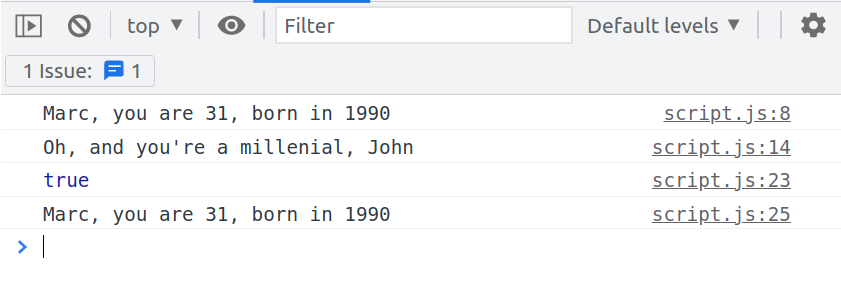# const - JS | lectureHow JavaScript Works Behind the Scenes

Scoping in Practice

This lecture will put everything we learned in the previous lesson on scoping in action in a semi-complex example to ensure that you get the concept.

We will start by writing a simple function that calculates a user's age called `calcAge`, which receives the birth year as a parameter and returns the result.

script.js
``````function calcAge(birthYear) {
const age = 2021 - birthYear;
return age;
}``````

The above `calcAge` function, as we learned in the last lecture, is defined in a global scope because it is in the top-level code, and it also creates its scope, which is going to be equivalent to the variable environment of its execution context.

Now let's create a global variable called `firstName`, to which I'm going to assign a value of `'Marc'`, and on the following line, let's call our `calcAge` function with the year `1990` as the parameter.

Before executing it, let's add a `console.log` in the `calcAge` function to log the `firstName` variable. This is what you should finally have:

script.js
``````function calcAge(birthYear) {
const age = 2021 - birthYear;
console.log(firstName);
return age;
}
const firstName = 'Marc';calcAge(1990);``````

From the above code, you can see that the firstName variable is not in the scope of the calcAge function; however, it is a global variable that we defined out of the calcAge function. Therefore, it will also be made available inside the calcAge function scope through the scope chain.

If we try to run or execute the above code in the browser, we will see Mark printed in the console, the firstName variable.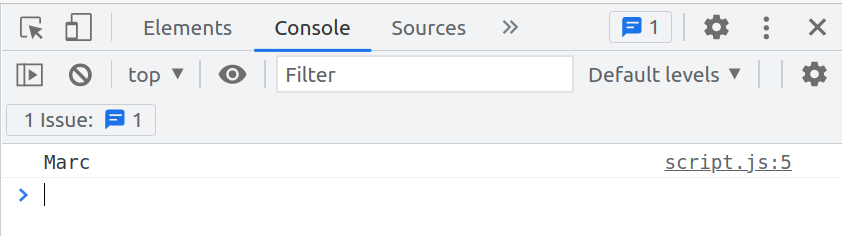To explain what just happened, when JavaScript executed the below line of code

script.js
``console.log(firstName);``

JavaScript did not find the `firstName` variable in the `calcAge` function scope. So it did a variable lookup, where it looked up in the scope chain to see if it found the variable there. The parent scope of the `calcAge` function is the global scope, and the `firstName` variable is in there; therefore, JavaScript could then use that.

Now, if we tried, for example, to log a variable that doesn't exist, say `lastName`, then JavaScript would also do a lookup, but it would not find that variable in the global scope, and therefore, we would get an error.

script.js
``````function calcAge(birthYear) {
const age = 2021 - birthYear;
// We will get an error: ReferenceError
console.log(lastName);  return age;
}

const firstName = 'Marc';
calcAge(1990);``````

Even though the `firstName` variable was defined after the `calcAge` function, that's not a problem. Remember that the code in the function is only executed once it's called. And so, that happens after the declaration of the `firstName` variable; and at this point in the code, the `firstName` variable is already in the global execution variable environment. So in the global scope, ready to be used.

Instead of just logging the `firstName` variable to the console, let's get rid of it, and let's create another function inside the `calcAge` function called `printAge` that will print a nice string to the console. And as you also already know, it will also create a new scope.

script.js
``````function calcAge(birthYear) {
// JS Code (JavaScript Code)
function printAge() {
// JS Code
}
}``````

Let's create an `output` variable, which will be the below string.

script.js
``````function calcAge(birthYear) {
const age = 2021 - birthYear;

function printAge() {    const output = `You are \${age}, born in \${birthYear}`;    console.log(output);  }
return age;
}``````

Now to actually see this result of our function, we need to, of course, call the function.

script.js
``````function calcAge(birthYear) {
const age = 2021 - birthYear;

function printAge() {
const output = `You are \${age}, born in \${birthYear}`;
console.log(output);
}

printAge();  return age;
}``````

If you check your browser, you will see that it works just fine.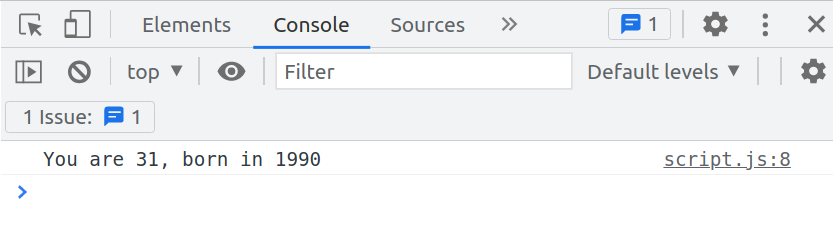We get the `age` that was calculated here:

script.js
``const age = 2021 - birthYear;``

And also the `birthYear` that was passed into the `calcAge` function.

Here again, we see the magic of the scope chain in action. Once again, as it is executing the `printAge` function, the engine is trying to access or find the `age` variable in the current scope. However, it cannot find it there, and so, therefore, it goes to the parent scope, where it will then find the `age` variable that we created.

The same is true for the `birthYear` variable because the parameter of a function works like normal variables for scoping.

Remember also that we said that the scope of a variable is the entire region of the code in which the variable is accessible.For example, the scope of the `age` variable is all the highlighted area in the `calcAge` function.

script.js
``````function calcAge(birthYear) {
const age = 2021 - birthYear;  function printAge() {    const output = `You are \${age}, born in \${birthYear}`;    console.log(output);  }  printAge();  return age;}``````

That is in the `calcAge` function, where it was defined, and then also in all the child scopes, .i.e. all the inner scopes.

On the other hand, `age` is not accessible outside the `calcAge` scope. We can prove that by trying to log the age variable out of the `calcAge` function.

script.js
``````function calcAge(birthYear) {
const age = 2021 - birthYear;

// JS Code
}

const firstName = 'Marc';
calcAge(1990);

console.log(age); // ReferenceError``````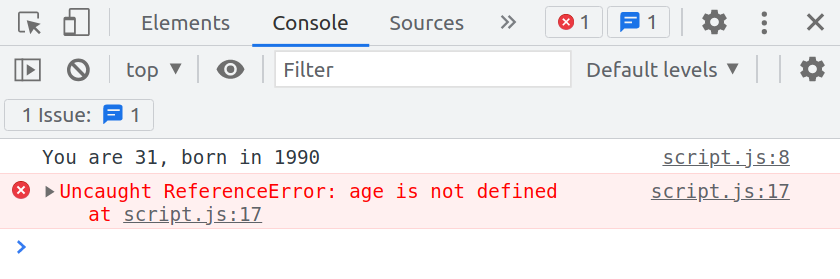That is because, as I mentioned, the scope chain is a one-way street. Only an inner scope can have access to the variables of its outer scope, but not the other way around.

So when doing `console.log(age)`, we are in the outer scope, and we cannot have access to the variables of a child scope, which in this case is the `calcAge` function. The same goes for functions too. We cannot call the `printAge` function out of the `calcAge` function for the same reason.

So if we try we get the below reference error.

script.js
``````function calcAge(birthYear) {
const age = 2021 - birthYear;

function printAge() {
// JS Code
}

// JS Code
}

const firstName = 'Marc';
calcAge(1990);

printAge(); // ReferenceError``````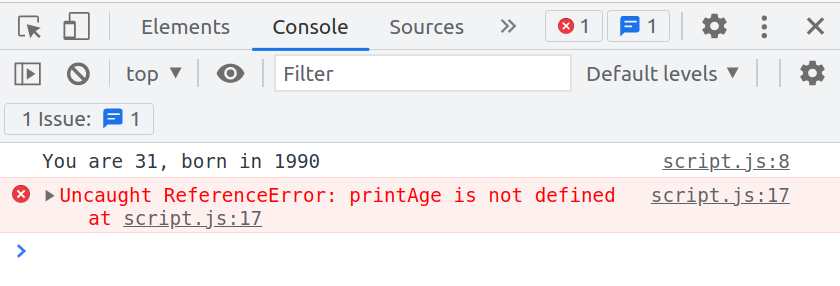This shows that in the global scope, we do not have access to any variables defined in any other scope.

Let's take it to the next level and include the `firstName` variable in our `output` string.

script.js
``````function printAge() {
const output = `\${firstName}, you are \${age}, born in \${birthYear}`;
console.log(output);
}``````

If you now run it in the browser, you can see that it does have access to the `firstName` variable. In this case, the engine is doing an even bigger or a longer variable lookup.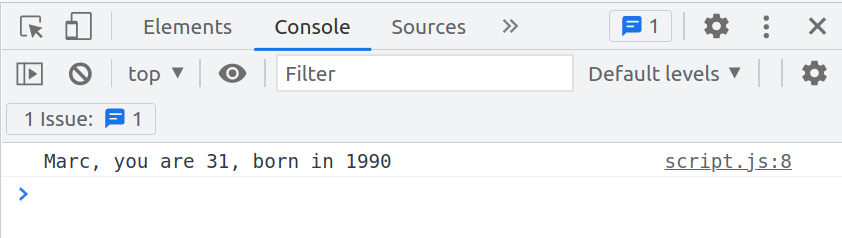Starting with the below code, we are in the `printAge` scope.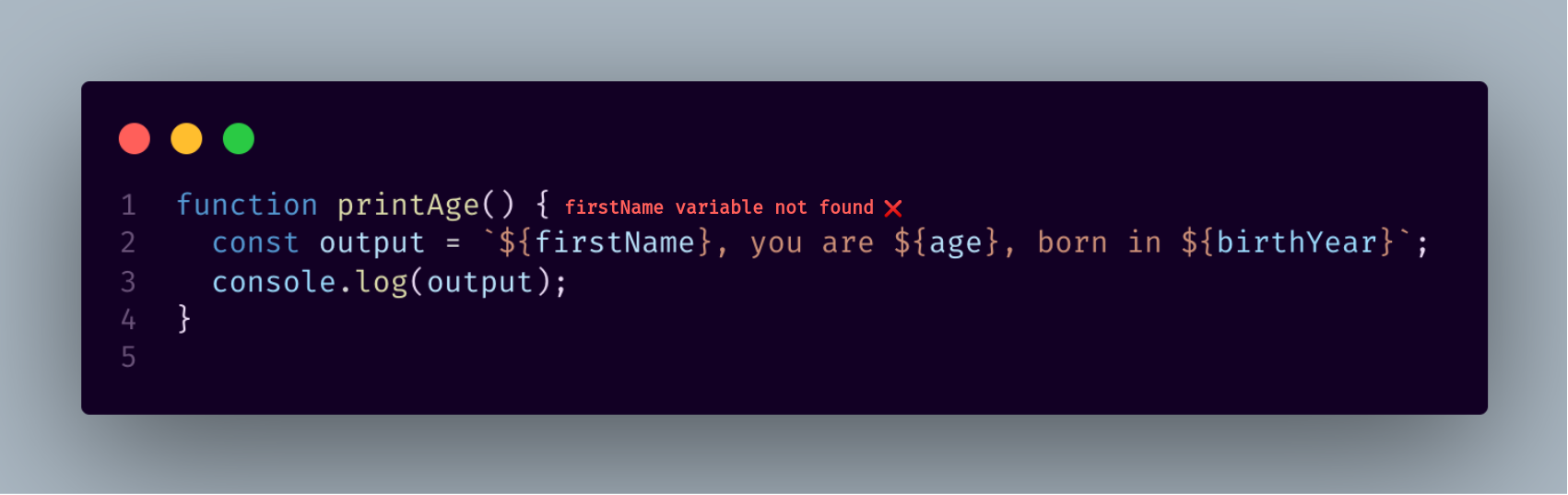JavaScript cannot find the variable in this scope, so it looks up in the scope chain, which is then the next step, the `calcAge` function.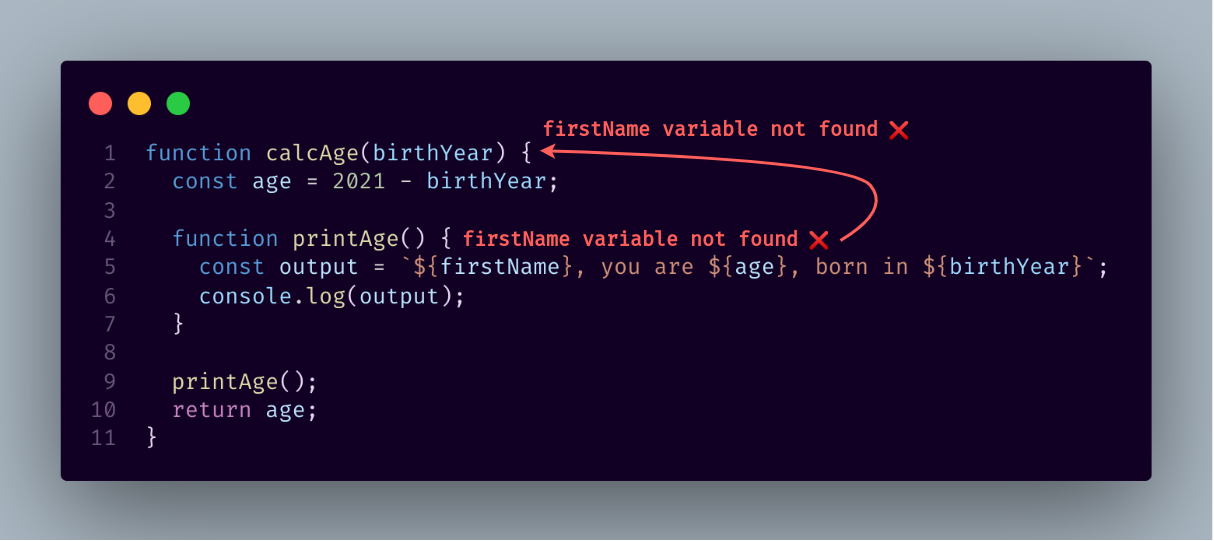However, it can also not find the `firstName` variable in this scope, and therefore it goes up even further into the global scope and finds it there.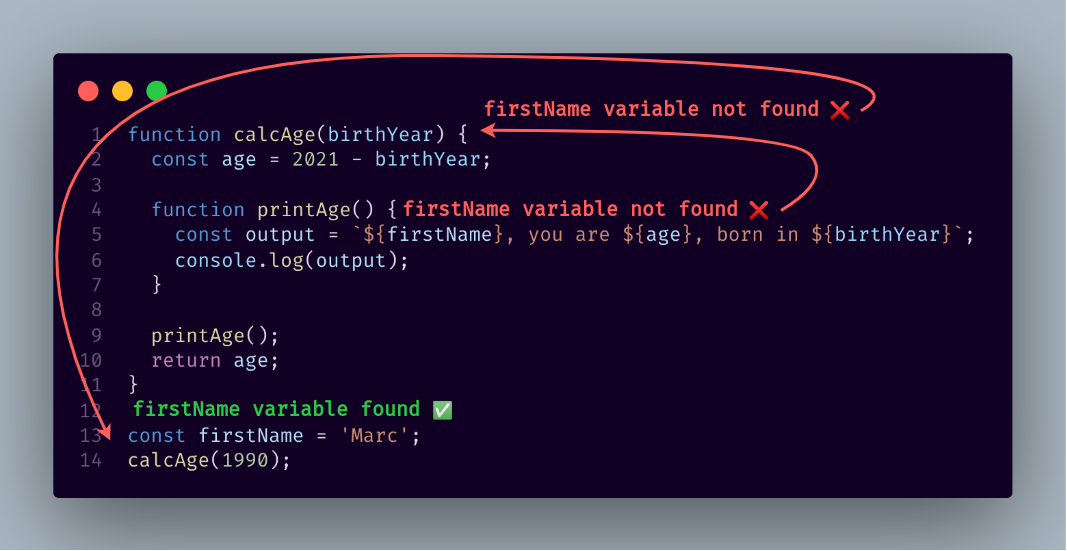I hope all of this made sense until this point. Let's go further and create a block scope in the `printAge` function. An `if` block to check if a person is a millennial

script.js
``````function calcAge(birthYear) {
const age = 2021 - birthYear;

function printAge() {
// JS Code
if (birthYear >= 1981 && birthYear <= 1996) {      const str = `Oh, and you're a millennial, \${firstName}`;      console.log(str);    }  }

// JS Code
}``````

We are adding even one more step here to the scope chain because the lookup for `firstName` is longer. If we run it again in the browser, you see that we are clearly a millennial, and our `firstName` `'Marc'` is still found from the outer scope.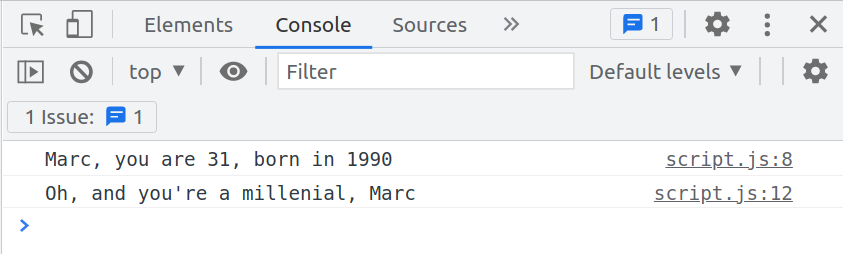Now, if we try to log the `str` variable outside the block scope, we get an error saying that `str` is not defined.

script.js
``````function calcAge(birthYear) {
const age = 2021 - birthYear;

function printAge() {
// JS Code
if (birthYear >= 1981 && birthYear <= 1996) {
const str = `Oh, and you're a millennial, \${firstName}`;
console.log(str);
}
console.log(str);  }

// JS Code
}``````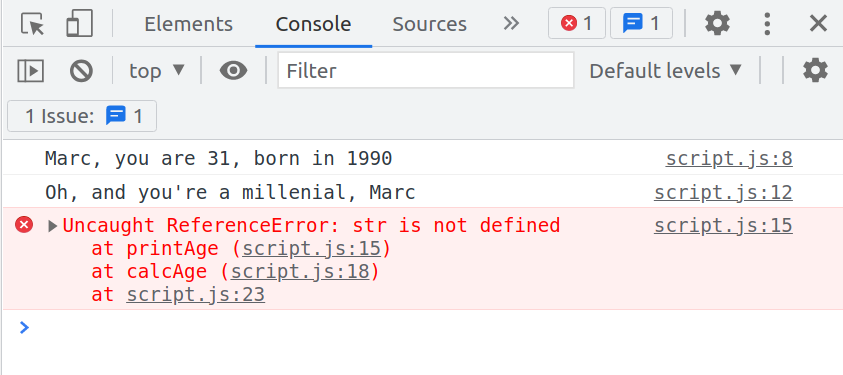Again, that's because const and let variables are block-scoped. They are available only inside the block in which they were created.

Let's now create another variable called `millennial`. This time it will be an old pre ES6 variable.

script.js
``````function calcAge(birthYear) {
const age = 2021 - birthYear;

function printAge() {
// JS Code
if (birthYear >= 1981 && birthYear <= 1996) {
var millennial = true;      const str = `Oh, and you're a millennial, \${firstName}`;
console.log(str);
}

console.log(millennial)
}

// JS Code
}``````

If we try to log it where we logged the str variable, JavaScript will find it.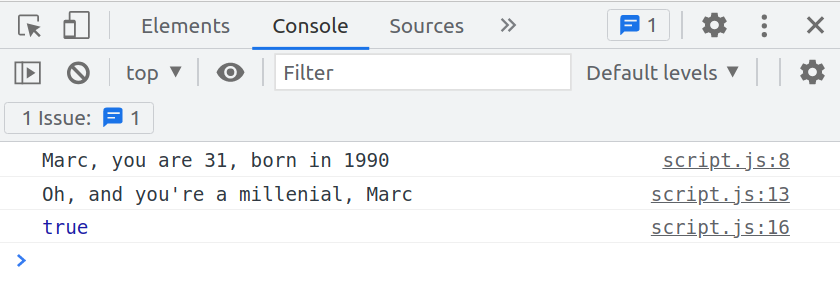That is because of the fact that `var` variables, i.e., variables declared with the `var` keyword, are function scoped. They simply ignore the block because they are not block-scoped at all. They're just function scoped.

As you can see above, we are still in the same function when logging the `millennial` variable. Right now, the scope of the `millennial` variable is the entire `printAge` function, no matter if it was declared inside of a block or not. Because again, `var` variables do not care about blocks at all. And so therefore, we can then access the `millennial` variable inside of its scope.

So keep that in mind as you use, or as you see, a variable declared with `var` because, as I said, on your own, you should probably not use this variable yourself. Always just use `const`, or `let`. But, if you are reading other codebases, or even working with older code, then keep in mind that this is how the var variable works.

Next up, let's prove that functions are also, in fact, block-scoped starting in ES6.

Let's start by creating a simple function in our if block that will add two values.

script.js
``````function calcAge(birthYear) {
const age = 2021 - birthYear;

function printAge() {
// JS Code
if (birthYear >= 1981 && birthYear <= 1996) {
var millennial = true;
//JS Code
function add(a, b) {        return a + b;      }    }
console.log(millennial);
}

// JS Code
}``````

Now, let's attempt to call it outside of the `if` block.

script.js
``````function calcAge(birthYear) {
const age = 2021 - birthYear;

function printAge() {
// JS Code
if (birthYear >= 1981 && birthYear <= 1996) {
var millennial = true;
//JS Code
return a + b;
}
}
console.log(millennial);

// JS Code
}``````

If we check our browser, we will see that we get `add` is not defined. That's because the scope of the add function is only the block in which it was defined. That is, the `if` block.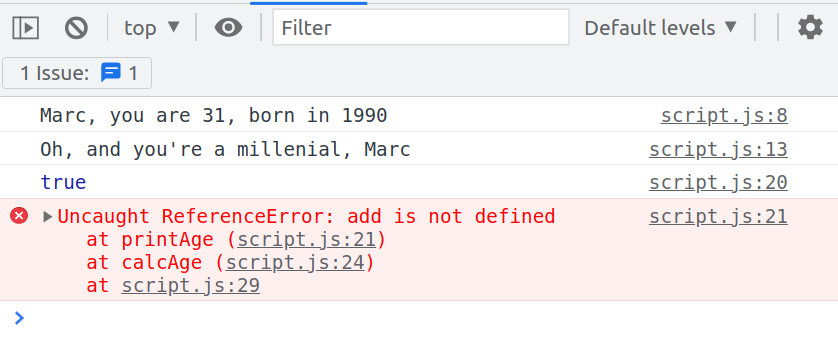This proves that functions are block-scoped. But remember that that is only true for strict mode. That's the mode that we're currently in.

But if we turn this off,

script.js
``//'use strict';``

You should now see add being called, and the result logged to the console. As I said at the beginning of this course, always stay in strict mode.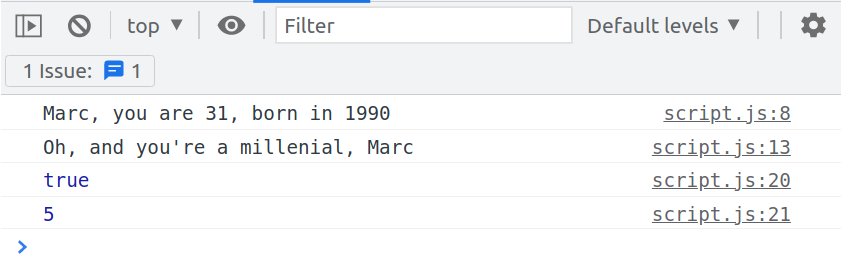Let's finish this lecture with an experiment. What do you think will happen if we declare a variable in the `if` block scope that already exists in a parent scope?

For example, let's say we declare another firstName variable in our if block,

script.js
``````function calcAge(birthYear) {
const age = 2021 - birthYear;

function printAge() {
// JS Code
if (birthYear >= 1981 && birthYear <= 1996) {
var millennial = true;
const firstName = 'John';      const str = `Oh, and you're a millennial, \${firstName}`;
console.log(str);

return a + b;
}
}
console.log(millennial);
}

// JS Code
}
const firstName = 'Marc';calcAge(1990);``````

What do you think the below string or variable `str` will look like now? Will it say `Marc` or `John`?

If we check our browser, we see that it says, `John`. That happens, because as always, JavaScript tries to look for the variable name in the current scope, and right now, it is in the current scope. So, since the `firstName` variable is in the current scope, JavaScript will then use that variable and not perform any variable lookup in the scope chain.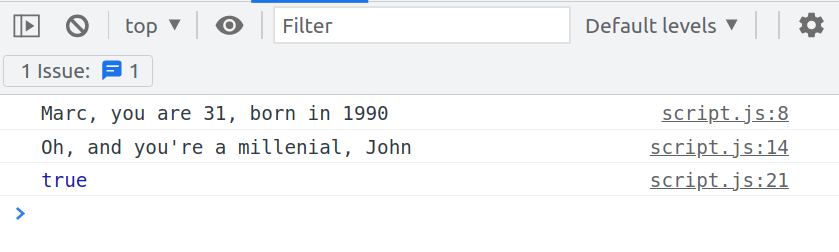So the scope chain isn't necessary if the variable that we're looking for is already in the current scope. And that's precisely the case right here.

Outside of the if block, the `firstName` variable will still be the one coming from the scope chain. That's why we still see Marc in the console coming from the `printAge` function, which is the parent scope of the `if` block scope.

Therefore from the above code, we can see that since the `firstName` variable is not in the current scope of the `printAge` function, JavaScript will make a variable lookup in the scope chain until it can find `firstName`, which is still `'Marc'` coming from the global scope.

In the if block scope, we created a new variable with the same name as another variable that we had already created in a parent scope. We will then have two `firstName` variables, and that's not a problem because they are entirely different variables defined in different scopes.

There is no problem with having repeated variable names. That's also the reason why you can have different functions with the same parameter names. Because again, each parameter is only defined in that scope of that function, and therefore, it's not a problem at all to have many functions with the same parameter names, in the same way, that it's not a problem to have functions with the same variable names inside of them.

Now, let's see what happens when we redefine a variable from a parent scope inside an inner scope. We are not creating a new variable; instead, we are simply reassigning the value of a variable.

In our `if` block, let assign another value to our `output` variable

script.js
``````function printAge() {
// Change cont to let
let output = `\${firstName}, you are \${age}, born in \${birthYear}`;  console.log(output);
if (birthYear >= 1981 && birthYear <= 1996) {
// JS Code
output = 'Something new';  }
console.log(millennial);

console.log(output);}``````

From the above code, we can see that we are in the printAge scope, and inside this scope, we have the `output` variable. The inner scope, which is the `if` block, redefines the `output` variable from the outer scope.

If we try to access the `output` variable, we get `'Something new'` in the console because we manipulated the existing variable inside a child's scope. That is, inside the `if` block scope.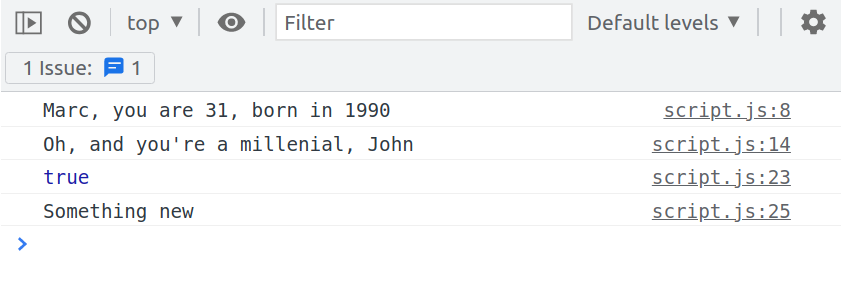We did not create a new variable. We simply redefined a variable that we accessed in the `if` block from the parent scope. If we created a new variable called `output` in the `if` block, we would have the same situation as before with `firstName`.

This would then be a completely different variable and would not affect the `output` from the outer scope. We will get back to the original output as we defined it in the parent scope if we try it.

script.js
``````function printAge() {

let output = `\${firstName}, you are \${age}, born in \${birthYear}`;
console.log(output);
if (birthYear >= 1981 && birthYear <= 1996) {
// JS Code
const output = 'Something new';  }
console.log(millennial);